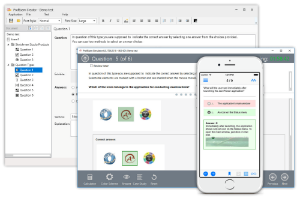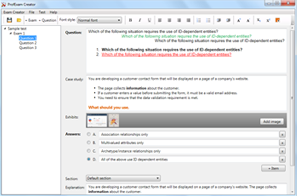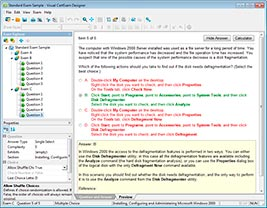## File Info

 Exam Upgrade to Java SE 8 OCP Number 1z0-813 File Name Upgrade to Java SE 8 OCP.prep4sure.1z0-813.2018-09-05.1e.36q.vcex Size 2.48 Mb Posted September 05, 2018 Downloads 51

### How to open VCEX & EXAM Files?

Files with VCEX & EXAM extensions can be opened by ProfExam Simulator.

Coupon: EXAMFILESCOM
With discount: 20%

## Demo Questions

Question 1
Given:and the code fragment:What is the result?

• A: A compilation error occurs.
• B: 2000.0
• C: 1500.0
• D: 0.0

Question 2
Which two codes correctly represent a standard language locale code? (Choose two.)

• A: u8
• B: fr
• C: es
• D: U8
• E: FR
• F: ES

Question 3
Given the code fragment:What is the result?

• A: Word: why what when
• B: Words: why Word: Why what Word: why what when
• C: Word: why Word: what Word: when
• D: Compilation fails.

Question 4
Given the code fragment:If exceptions occur when closing the FileWriter object and when retrieving the JString class object, which exception object is propagated up to the caller of the processFile method?

• A: java.lang.Exception
• B: java.io.IOException
• C: java.lang.ClassNotFoundException
• D: java.lang.NoSuchClassException

Question 5
Given:What is the result when you compile the code and execute the command:
java MyApp kava

• A: kava
• B: Kava
• C: kawa
• D: Kawa
• E: An exception is thrown at runtime.

Question 6
Given the code fragment:andWhich code fragment could you see to refactor the code from line 4 to 8 to use a Lambda expression?

• A: Vehicle v = new Vehicle(int speed) { System.out.print(“Fly at “ + speed); );
• B: Vehicle v = int speed -> System.out.print(“Fly at “ + speed);
• C: Vehicle v = (int speed) -> System.out.print(“Fly at “ + speed);
• D: Vehicle v = speed -> {System.out.print(“Fly at “ + speed) };

Question 7
Given the code fragment:What is the result?

• A: 0 0
• B: 1 2
• C: 1 4
• D: A compilation error occurs

Question 8
Given the incomplete pseudo-code for a fork/join framework application:And given the missing methods:
process, submit, and splitInHalf
Which three insertions properly complete the pseudo-code? (Choose three.)

• A: Insert process at line Z.
• B: Insert process at line X.
• C: Insert submit at line X.
• D: Insert splitInHalf at line X.
• E: Insert process at line Y.
• F: Insert splitInHalf at line Y.
• G: Insert submit at line Z.

Question 9
Given the code fragment:Which code fragment, when inserted at line n1, enables the code to print the count of string elements whose length is greater than three?

• A: listVal.stream().filter(x -> x.length() > 3).count()
• B: listVal.stream().map(x -> x.length() > 3).count()
• C: listVal.stream().peek(x -> x.length() > 3).count().get()
• D: listVal.stream().filter(x -> x.length() > 3).mapToInt(x -> x).count()

Question 10
Given the code fragments:andWhat is the result?

• A: A compilation error occurs at line n2.
• B: The program prints Run… and throws an exception.
Run…
• C: Call…
• D: A compilation errors occurs at line n1.

CONNECT US##### PROFEXAM WITH A 20% DISCOUNT

You can buy ProfExam with a 20% discount..

HOW TO OPEN VCEX AND EXAM FILES

Use ProfExam Simulator to open VCEX and EXAM filesHOW TO OPEN VCE FILES

Use VCE Exam Simulator to open VCE files